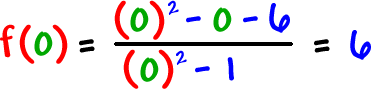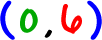These are easy!

Y-INTERCEPTS:  Where the graph crosses the y-axis

OK, think about it...  When you are on the y-axis, what is your
x value?The x value is 0.So, find the y-intercept...Set x = 0!

That's the same as finding f(0).

Check it out:So, the y-intercept for this guy is the pointTRY IT:

Find the y-intercept of